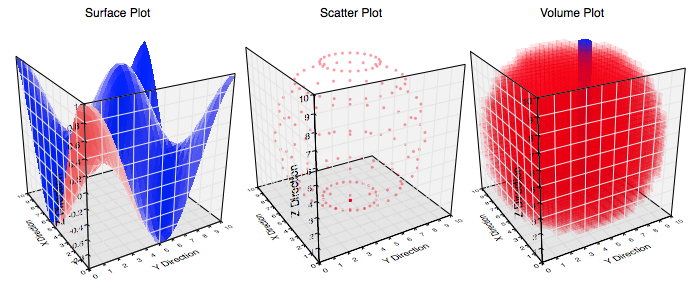Graph IDE ► 3D Data Graphics

A three dimensional data graphic is a graphic which is defined by three dimensional points and has point-wise graphic representations. When a 3D graphic is created it is always accompanied by a graph. That is because the 3D graphic can not be represented on a page, it needs some sort of projection which is defined by the graph.

Examples of a three dimensional data graphics are shown below.The following is a brief list and definition of three dimensional data graphics:

 Section Description Graph The graph on which 3D data graphics are made. Point Map A Point Map is a regular grid of z-values. The z-values are represented as the z-coordinate value thus producing the three dimensional effect. Scatter A scatter is a set of 3D points. Volume A volume is a 3D density plot made from a sequence of regularly placed density values and represented by cubes.